International
Tables for
Crystallography
Volume B
Reciprocal space
Edited by U. Shmueli

International Tables for Crystallography (2006). Vol. B. ch. 2.3, pp. 244-245   | 1 | 2 |

## Section 2.3.3.5. Interpretation of isomorphous difference Pattersons

M. G. Rossmanna* and E. Arnoldb

aDepartment of Biological Sciences, Purdue University, West Lafayette, Indiana 47907, USA, and  bCABM & Rutgers University, 679 Hoes Lane, Piscataway, New Jersey 08854-5638, USA
Correspondence e-mail:  mgr@indiana.bio.purdue.edu

#### 2.3.3.5. Interpretation of isomorphous difference Pattersons

| top | pdf |

Difference Pattersons have usually been manually interpreted in terms of point atoms. In more complex situations, such as crystalline viruses, a systematic approach may be necessary to analyse the Patterson. That is especially true when the structure contains noncrystallographic symmetry (Argos & Rossmann, 1976). Such methods are in principle dependent on the comparison of the observed Patterson,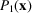, with a calculated Patterson,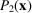. A criterion,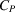, based on the sum of the Patterson densities at all test vectors within the unit-cell volume V, would be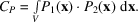can be evaluated for all reasonable heavy-atom distributions. Each different set of trial sites corresponds to a differentPatterson. It is then easily shown that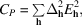where the sum is taken over all h reflections in reciprocal space,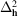are the observed differences andare the structure factors of the trial point Patterson. (The symbol E is used here because of its close relation to normalized structure factors.)

Let there be n noncrystallographic asymmetric units within the crystallographic asymmetric unit and m crystallographic asymmetric units within the crystal unit cell. Then there are L symmetry-related heavy-atom sites where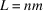. Let the scattering contribution of the ith site haveand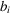real and imaginary structure-factor components with respect to an arbitrary origin. Hence, for reflection h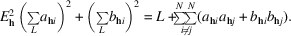Therefore,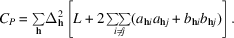But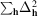must be independent of the number, L, of heavy-atom sites per cell. Thus the criterion can be re-written as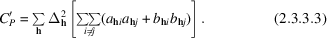More generally, if some sites have already been tentatively determined, and if these sites give rise to the structure-factor components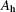and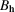, then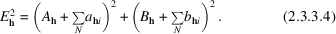Following the same procedure as above, it follows that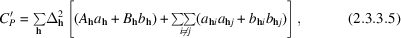where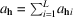and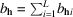.

Expression (2.3.3.5)will now be compared with the `feedback' method (Dickerson et al., 1967, 1968) of verifying heavy-atom sites using SIR phasing. Inspection of Fig. 2.3.3.4shows that the native phase, α, will be determined as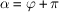(ϕ is the structure-factor phase corresponding to the presumed heavy-atom positions) when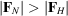and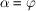when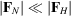. Thus, an SIR difference electron density,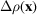, can be synthesized by the Fourier summation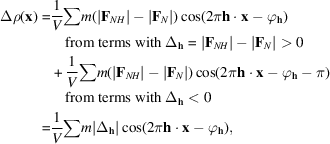where m is a figure of merit of the phase reliability (Blow & Crick, 1959; Dickerson et al., 1961). Now,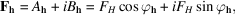whereandare the real and imaginary components of the presumed heavy-atom sites. Therefore,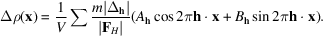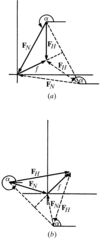Figure 2.3.3.4 | top | pdf |The phase α of the native compound (structure factor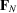) is determined either as being equal to, or 180° out of phase with, the presumed heavy-atom contribution when only a single isomorphous compound is available. In (a) is shown the case when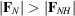and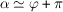. In (b) is shown the case when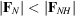and, where ϕ is the phase of the heavy-atom structure factor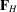.

If this SIR difference electron-density map shows significant peaks at sites related by noncrystallographic symmetry, then those sites will be at the position of a further set of heavy atoms. Hence, a suitable criterion for finding heavy-atom sites is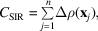or by substitution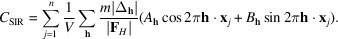But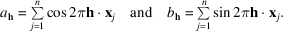Therefore,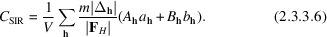This expression is similar to (2.3.3.5)derived by consideration of a Patterson search. It differs from (2.3.3.5)in two respects: the Fourier coefficients are different and expression (2.3.3.6)is lacking a second term. Now the figure of merit m will be small whenever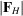is small as the SIR phase cannot be determined well under those conditions. Hence, effectively, the coefficients are a function of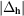, and the coefficients of the functions (2.3.3.5)and (2.3.3.6)are indeed rather similar. The second term in (2.3.3.5)relates to the use of the search atoms in phasing and could be included in (2.3.3.6), provided the actual feedback sites in each of the n electron-density functions tested by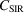are omitted in turn. Thus, a systematic Patterson search and an SIR difference Fourier search are very similar in character and power.

### ReferencesArgos, P. & Rossmann, M. G. (1976). A method to determine heavy-atom positions for virus structures. Acta Cryst. B32, 2975–2979.Google ScholarBlow, D. M. & Crick, F. H. C. (1959). The treatment of errors in the isomorphous replacement method. Acta Cryst. 12, 794–802.Google ScholarDickerson, R. E., Kendrew, J. C. & Strandberg, B. E. (1961). The crystal structure of myoglobin: phase determination to a resolution of 2 Å by the method of isomorphous replacement. Acta Cryst. 14, 1188–1195.Google ScholarDickerson, R. E., Kopka, M. L., Varnum, J. C. & Weinzierl, J. E. (1967). Bias, feedback and reliability in isomorphous phase analysis. Acta Cryst. 23, 511–522.Google ScholarDickerson, R. E., Weinzierl, J. E. & Palmer, R. A. (1968). A least-squares refinement method for isomorphous replacement. Acta Cryst. B24, 997–1003.Google Scholar